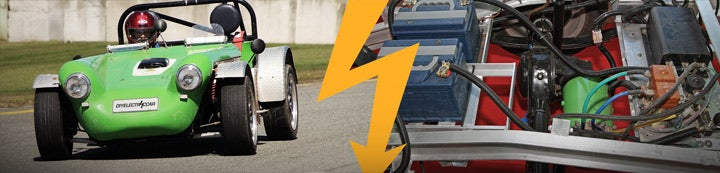1 - 1 of 1 Posts

#### EVDL List

·
##### Registered
Joined
·
70 Posts
Discussion Starter · ·
Re: [EVDL] Converting from watt-hours/mi. to mi/gal & back

But you're comparing two different things - the electricity that you
put into the battery and the energy you get out of the gas.

In that calculation, you're saying that mpg before equals mpg after
and using that to find the conversion.

My way of doing it (excluding the efficiencies from the calculation)
gives you power in to range out, which is a measure of the actual
efficiency of the vehicles. I'm not saying that putting 36kWh of gas
into your ICE is the same as putting 36kWh of electricity into your
EV; I'm saying that it takes 1100Wh of chemical energy into the gas
tank to go one mile, as opposed to 300Wh of electricity into the
battery to go one mile.

Your way, dividing miles per gallon of the gas vehicle by miles per
kWh of the EV, is more useful for comparing the cost of gas to the
cost of electricity. It says "if I'm putting 10 gallons of gas into
the ICE, I could drive the same distance by putting 100kWh of
electricity into the EV" and lets you multiply by the cost per
gallon/cost per kWh to find running costs. However, using this method,
when trying to find the miles per gallon equivalent, you'll always get
the same number (32 mpg). This makes mpg useless for comparing the
efficiency of the vehicles.

So it looks like there's two different ways to calculate this: using
all the energy contained in the gas (36kWh) lets you compare the
efficiencies of the vehicles, while using only the useful energy
gotten out of the gas (~10kWh) lets you compare the cost of gas with
the cost of electricity.

Since the OP was looking for a comparison of the efficiencies of the
vehicles, I assumed he wanted the equivalence that didn't cancel out
the efficiency, not the equivalence that will always give you 32 mpg.

(Try it: if you use your factor, just under 10kWh/gallon, then his
Civic as an electric gets just under 33 mpg (32), just like the gas
one. This formula always gives the same mpg for gas and electric, as
that's what it's based on. That's good if you're trying to find the
electricity costs, but bad if you're trying to find efficiencies.)

-Morgan

_______________________________________________
For subscription options, see
http://lists.sjsu.edu/mailman/listinfo/ev

1 - 1 of 1 Posts